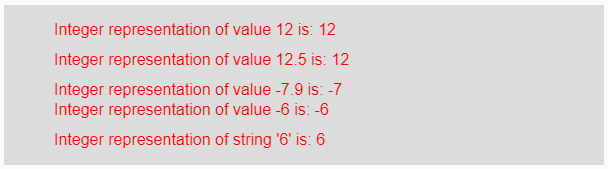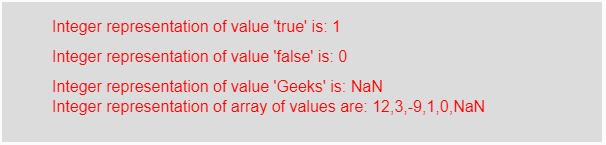# p5.js | int() function

The int() function in p5.js is used to convert the given boolean, integer and float value into its integer representation.

Syntax:

`int(ns)`

or

`int(n, radix)`

Parameters: This function accepts three types of parameter which are listed below:

• n: It stores the value which need to converts into integer. The possible value of n are string, boolean or number.
• radix: It store the radix part to which the number is converted.
• ns: It stores different types of element into the array.

Return Value: It returns the converted integer representation.

Below programs illustrate the int() function in p5.js:

Example 1: This example uses int() function to convert input value into its integer value.

 `function` `setup() {  ` `  `  `    ``// Creating Canvas size ` `    ``createCanvas(600, 160);  ` `}  ` `  `  `function` `draw() {  ` `      `  `    ``// Set the background color  ` `    ``background(220);  ` `    `  `    ``// Initializing some strings ` `    ``let Value1 = 12; ` `    ``let Value2 = 12.5; ` `    ``let Value3 = -7.9; ` `    ``let Value4 = -6; ` `    ``let Value5 = ``"6"``; ` `    `  `    ``// Calling to float() function. ` `    ``let A = int(Value1); ` `    ``let B = int(Value2); ` `    ``let C = int(Value3); ` `    ``let D = int(Value4); ` `    ``let E = int(Value5); ` `      `  `    ``// Set the size of text  ` `    ``textSize(16);  ` `      `  `    ``// Set the text color  ` `    ``fill(color(``'red'``));  ` `    `  `    ``// Getting integer representation ` `    ``text(``"Integer representation of value 12 is: "` `+ A, 50, 30); ` `    ``text(``"Integer representation of value 12.5 is: "` `+ B, 50, 60); ` `    ``text(``"Integer representation of value -7.9 is: "` `+ C, 50, 90); ` `    ``text(``"Integer representation of value -6 is: "` `+ D, 50, 110); ` `    ``text(``"Integer representation of string '6' is: "` `+ E, 50, 140); ` `}   `

Output:Example 2: This example uses int() function to convert input value into its integer value.

 `function` `setup() {  ` `  `  `    ``// Creating Canvas size ` `    ``createCanvas(600, 140);  ` `}  ` `  `  `function` `draw() {  ` `      `  `    ``// Set the background color  ` `    ``background(220);  ` `    `  `    ``// Initializing some values ` `    ``let Value1 = ``true``; ` `    ``let Value2 = ``false``; ` `    ``let Value3 = ``"Geeks"``; ` `    ``let Value4 = [12, 3.6, -9.8, ``true``, ``false``, ``"Geeks"``]; ` `    `  `    ``// Calling to int() function. ` `    ``let A = int(Value1); ` `    ``let B = int(Value2); ` `    ``let C = int(Value3); ` `    ``let D = int(Value4); ` `      `  `    ``// Set the size of text  ` `    ``textSize(16);  ` `      `  `    ``// Set the text color  ` `    ``fill(color(``'red'``));  ` `    `  `    ``// Getting integer representation ` `    ``text(``"Integer representation of value 'true' is: "` `+ A, 50, 30); ` `    ``text(``"Integer representation of value 'false' is: "` `+ B, 50, 60); ` `    ``text(``"Integer representation of value 'Geeks' is: "` `+ C, 50, 90); ` `    ``text(``"Integer representation of array of values are: "` `+ D, 50, 110); ` `}   `

Output:Note: From the above program, if the value of the parameter is number then it returns output as integer representation, for false it returns 0 and for true it returns 1 and for string values, it returns NaN i.e, not a number.

Reference: https://p5js.org/reference/#/p5/int

My Personal Notes arrow_drop_upCheck out this Author's contributed articles.

If you like GeeksforGeeks and would like to contribute, you can also write an article using contribute.geeksforgeeks.org or mail your article to contribute@geeksforgeeks.org. See your article appearing on the GeeksforGeeks main page and help other Geeks.

Please Improve this article if you find anything incorrect by clicking on the "Improve Article" button below.

Improved By : ManasChhabra2

Article Tags :

Be the First to upvote.

Please write to us at contribute@geeksforgeeks.org to report any issue with the above content.